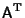## Antisymmetric Matrix

An antisymmetric matrix is a Matrix which satisfies the identity(1)

whereis the Matrix Transpose. In component notation, this becomes(2)

Letting, the requirement becomes(3)

so an antisymmetric matrix must have zeros on its diagonal. The generalantisymmetric matrix is of the form(4)

Applyingto both sides of the antisymmetry condition gives(5)

Any Square Matrix can be expressed as the sum of symmetric and antisymmetric parts. Write(6)

But(7)(8)

so(9)

which is symmetric, and(10)

which is antisymmetric.